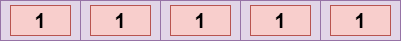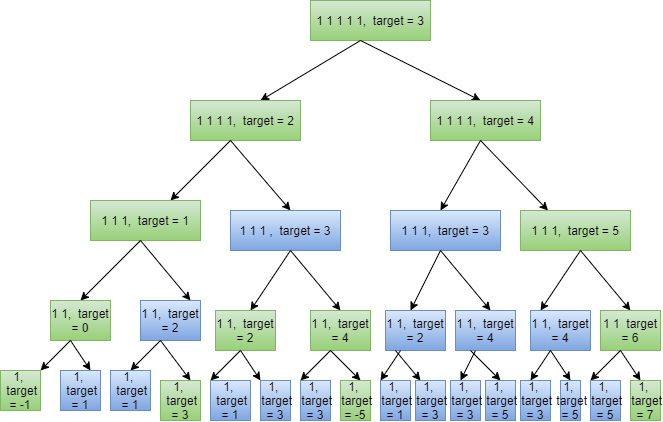# Target Sum

## Introduction

The problem we are going to face in this blog is the target sum which is again a popular question of data structure. By reading the name of the problem, what do you think this problem would be?

Yeah, you are right; we will be provided with a target for which we have to check whether the target sum is reached or not.

Let's understand the problem more clearly through the problem statement. So are you ready for this?

## Problem Statement

The problem statement in your interviews will be given like this: You are given an array of integers and a number target. You want to build an expression out of an array by adding one of the symbols '+' and '-' before each integer in an array, and then by concatenating all the integers, you want to achieve a target. You will be asked to return the number of different expressions that you can build, which evaluates to the target.

So let’s see an example to understand the problem statement better:

You are having array nums:Suppose the target given to you is 3. Now let’s see the ways in which we can assign symbols to the array elements to get target as 3:-

• -1 + 1 + 1 + 1 + 1 = 3
• +1 - 1 + 1 + 1 + 1 = 3
• +1 + 1 - 1 + 1 + 1 = 3
• +1 + 1 + 1 - 1 + 1 = 3
• +1 + 1 + 1 + 1 - 1 = 3

So these are the five ways you can assign symbols to the array of elements. So your output will be 5. Since we have understood the problem clearly, now is the time to move towards its solution.

## Approach 1: Using Recursion

For every array element, we have a choice of whether to include it with the positive sign or whether to include it with a negative sign. Remember, whenever you have choices, this is an indication that the question can be solved with recursion

Note: For more details, you can also refer to the Free content of Recursion.

So let’s see the steps and what exactly the approach should be:

Step1: First, decide on the base case. Suppose the array given to you is of zero sizes, and the target given to you is also zero. Then your output will be 1 because you have one way to make a zero target. Another situation can be that the size of the array is zero, but the target is non-zero. Now there is no way to achieve the target, so your output will be zero.

Step 2: Now, let’s make the recursion calls. Two recursion calls will be made since we have two choices on every element:

• We will take the element at zero index with a positive sign. So the target we are left with is target - arr.
• We will take the element at zero index with a negative sign. So the target we are left with is target + arr.

Step 3: Now return the sum of answers brought by both recursion calls, and you are done. Yes! That is simple.

Now trying coding it on our own, and then I’ll be sharing my code:

### Complexity Analysis

Time Complexity: O(2 ^ N) where ‘N’ refers to the number of elements in the array. Since we have two choices at every element, that’s why we have exponential time complexity.

Space Complexity: O(N). Due to recursion, we are consuming space in the recursive call stack.

In the above method, we are making several overlapping recursive calls. Due to this the time complexity we have achieved in the above solution is not good.The boxes marked in blue color denote the overlapping recursive calls. You can see that we are making so many overlapping calls which are costing us time and complexity. So let’s try improving it with memoization.

## Approach 2: Using Memoization

In the previous method, the main problem was that we were making several overlapping recursive calls. So here, what we will be doing is that we will store the output of each call in an array, and if the output of some calls is already present in this array, we will not be making that recursive call.

The code will be the same as that of approach 1. Here the only difference is that make a 2-D array named ‘mem’ that will store the result of each call. Now rows of this 2D array will signify the start of the array, and columns will be signifying targets.

Target can be any integer, so the question in your head will be how will I decide the number of columns in my array. Good Question! The answer is that we will be using an unordered map here to store different targets. You will understand it better by going through the below code:

### Complexity Analysis:

Time Complexity: O(T * N), where ‘N’ refers to the size of the array and ‘T’ refers to the sum of the array. The memo of size (T * N) has been filled only once; that’s why we have obtained this time complexity which is quite better than the previous approach.

Space Complexity: O(T * N). The depth of the recursion tree can go up to ‘N’.

Now is the time to move to the Dynamic Programming Approach. Remember, before moving to the DP approach, you should always try its recursive and memoized approach first. It will help you a lot in making a good impression in your interviews.

## Approach 3: Using 2-D Dynamic Programming

The idea behind this approach is as follows. Suppose we can find out the number of times a particular sum, say ‘sum_i’ is possible up to a specific index, say ‘i’ in the given array. We can now determine the number of times the sum, sum_i + nums[i], and sum_i - nums[i] can occur easily using the previous result. Let’s understand this approach clearly in steps:

Step1: Make a 2-D DP array where,

• Row refers to the size of the array,
• The column refers to the sums we can achieve upto that index.
• In this array on each cell, we will be storing the number of ways possible to achieve that sum.

Step 2: Now, when the size of the array is 0, and the sum we want to achieve is zero, the number of ways for it will be 1. So just put dp = 1.

Step 3: Now, for each array element, the sums possible upto that index will be:-

• The sums possible upto the previous index + current array element
• The sums possible upto the previous index - current array element

Step 4: Keep filling this array until the size of the array becomes ‘N’.

Step 5: After these 4 steps, you will have your answer placed at dp[N][target].

Now I’ve discussed all the steps. Please try to code it on your own only then you will be able to rock in your interviews.

### Complexity Analysis

Time Complexity: O(T * N), where ‘N’ refers to the size of the array and ‘T’ refers to the sum of the array. The memo of size (T * N) has been filled only once; that’s why we have obtained this time complexity which is quite better than the previous approach.

Space Complexity: O(T * N) because the memo array contains (T * N) elements.

## Approach 4: Using 1-D Dynamic Programming

In the previous approach, we have used 2-D Dp in order to solve this problem, but there is one more optimized approach where we can reduce space complexity. So let’s discuss this approach now:

We will be using unordered maps here, and in unordered_map, the key value will signify the sum possible up to that current index, and the value will signify the number of ways to achieve that sum or target. It’s almost the same as Approach 3; the only difference is that since we need sums of the previous index only to fill the current index, there is no need to store results of all elements other than the previous. You will get a better idea after going through the below steps:-

Step 1. Take two unordered maps. One will store the sums possible up to the current index, and the other one will store the sums possible upto the previous index.

Step 2: Now for storing the number of ways to achieve sums or targets possible up to the current index, we just need to iterate the map storing sums possible up to the previous index.

And keep repeating step 2 until you reach the end of the given input vector. Let’s see how this approach can be coded:

### Complexity Analysis

Time Complexity: O(T * N), where ‘N’ refers to the size of the array and ‘T’ refers to the sum of the array. The memo of size (T * N) has been filled only once; that’s why we have obtained this time complexity which is quite better than the previous approach.

Space Complexity: O(T) where ‘T’ refers to the sum of the array. Two unordered maps are used, due to which we are getting this time complexity.

### What is the target sum Problem?

In the target sum problem, you have to find ways to add + or sign to each element so that you can achieve your target on solving that expression.

### Can I solve the target sum problem in O(N) time complexity?

No, You can’t because even the most optimized approach that is the one which I have discussed as approach 3 in this blog, is using O(T * N)

## Conclusion

This blog has discussed four approaches for the target sum problem. We have seen the time and space complexity of all these processes. Remember, you can make a good impression in an interview if you approach a problem in the same manner that I have done in this blog that first shares the recursive approach, then moves on to optimizing it as a memoized solution, and then finally present the dynamic programming solution. Remember, the more you practice, the more quickly you will be in getting logic to a question, so refer to the Top Dynamic programming QuestionsTop Recursion and Backtracking questions, etc.

Refer to our guided paths on Codestudio to learn more about Data Structures and AlgorithmsCompetitive Programming, etc. Also, enroll in Dynamic Programming and Recursion courses to crack the big tier companies.

If you feel that this blog has added some value to your knowledge, then please do share it with your friends. Happy Coding!